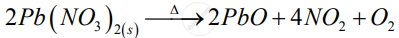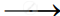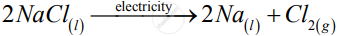Share

# Decomposition Reactions Require Energy Either in the Form of Heat Or Light Or Electricity for Breaking Down the Reactants. Write One Equation Each for Decomposition Reactions Where Energy is Supplied in the Form of Heat, Light and Electricity - CBSE Class 10 - Science

ConceptChemical Reactions Types of Chemical Reactions - Decomposition

#### Question

Decomposition reactions require energy either in the form of heat or light or electricity for breaking down the reactants. Write one equation each for decomposition reactions where energy is supplied in the form of heat, light and electricity

#### Solution

Those reactions in which a compound splits up into two or more simpler substances are known as decomposition reactions.

1)2)

2AgCl_((s)) + Sunlight2Ag_(s) + Cl_(2(g))

3)Is there an error in this question or solution?

#### Video TutorialsVIEW ALL 

Solution Decomposition Reactions Require Energy Either in the Form of Heat Or Light Or Electricity for Breaking Down the Reactants. Write One Equation Each for Decomposition Reactions Where Energy is Supplied in the Form of Heat, Light and Electricity Concept: Chemical Reactions - Types of Chemical Reactions - Decomposition.
S